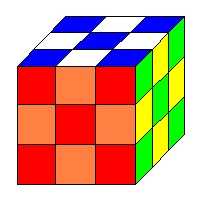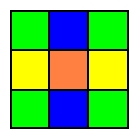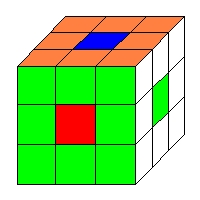Rubik's Cube Groups

If we consider all sequences of moves on a Rubik's Cube we notice the following:
• One sequence followed by another sequence is a sequence:  Closure.
• Followed by is associative.
• The do nothing sequence does nothing:  Identity.
• Every sequence of moves can be done backwards and therefore undone: Inverses.
This makes the set of all sequences of moves on a Rubik's Cube a group.  However since a sequence of moves can be of any length (you can do RLU over and over until the cows come home) let's cut it down to a more manageable and useful set.  Two sequences will be considered equivalent if they have the same final effect on the cube.  We'll call the final effect of a sequence of moves a process.  Thus a process is a possible permutation of the cubelets together with any twists of the corners or flips of the edges.

One of the first questions we ask is, How many processes are there in the group of the cube?  In other words, what is the order of the group of the cube.  We can solve this question by counting the permissible permutations of edges and the permissible permutations of corners.

There are 8 corner cubelets and 12 edge cubelets.  Each corner cubelet can have three possible orientations ("twists") and each edge cubelet can have two possible orientations ("flips").  Thus the possible states of the cube can be no more than

Upper Bound on Order of Cube Group = (8!)(12!)(38)(212)

However, as we have seen in the previous chapter not all of these configurations are reachable from the initial (solved) state.  There we learned that only 1/12 of all conceivable states are actually reachable so to get the order of the cube we must divide the upper bound given above by 12

Order of the Cube Group = (8!)(12!)(38)(212) ÷ 12

This is a rather large number:  43,252,003,274,489,856,000 (or a little over 43 quintillion).  At the rate of 1,000,000 configurations per second it would take well over a million years to run through them all.  From this we can safely conclude that coupling random twiddling with patience is a poor strategy for solving the cube.

One of the first things we notice is that the cube group is not Abelian. F  followed by  R is not the same as R followed by F. ( To simplify the notation we will leave out the term "followed by" and simply juxtapose the moves in a sequence.)  To have some simpler fun we'll look at some of the easier subgroups of the cube group.

The F subgroup

This subgroup consists of all possible processes that are the result of turning only the front face F.  This has the disadvantage of being a very boring subgroup whose only elements are E (the identity), F, F2 and F-1.  This is just the Attayun--HOOT group that we've seen before in various disguises.  Of course there is nothing special about F.  There are other copies of the Attayu--HOOT group using other faces or even central slices.

The SLICE SQUARED subgroup

This is about the simplest non-trivial subgroup of the cube.  It consists of all those processes that are the result of limiting ourselves to the following moves:  X = (R2L2), Y= (U2D2) and Z = (F2B2).  This subgroup gets its name from the fact that each move is equivalent to moving a central "slice" by 180°.  Since a move is 90° a move of 180° is the same as a normal move followed by itself or "squared."

One of the first things we notice about this group is that it's Abelian; XY has the same effect on the cube as YX etc.  Also we notice that XYZ applied to a solved cube results in a cute "checkerboard" pattern:The "Checkerboard"

Since X, Y and Z are not only commute but are their own inverses we can write out all the elements of the slice squared group: E (the identity), X, Y, Z, XY, XZ, YZ, and XYZ.  This group has the Cayley Table given here:

 E X Y Z XY XZ YZ XYZ E E X Y Z XY XZ YZ XYZ X X E XY XZ Y Z XYZ YZ Y Y XY E YZ X XYZ Z XZ Z Z XZ YZ E XYZ X Y XY XY XY Y X XYZ E YZ XZ Z XZ XZ Z XYZ X YZ E XY Y YZ YZ XYZ Z Y XZ XY E X XYZ XYZ YZ XZ XY Z Y X E

Cayley Table of the SLICE SQUARED Subgroup

The SLICE subgroup

This subgroup is large enough to be challenging to the beginning cubologist but not too big to be overwhelming.  It consists of all processes generated by the moves X = (RL-1), Y= (UD-1) and Z = (FB-1).  This group gets its name from the fact that each of the allowed moves is equivalent to a move of a middle "slice."  In the slice group the patterns on each face exhibit a certain symmetry.  The corner facelets of each face are always the same color.  Also, opposite edge facelets of each face are the same color.  This gives a pattern similar to this:Pattern in the SLICE group

One cute pattern in the slice group is formed by the move sequence LR-1BF-1UD-1LR-1
This pattern goes by the name of "dots"Dots

Sanity Check:  A would-be cubologist tells you that the SLICE group has exactly 108 elements.  How do you know, imediately, that this is incorrect?
...
...
...
...
...
Solution to Sanity Check:  The SLICE group contains the SLICE SQUARED group as a subgroup.  Thus the order of the SLICE SQUARED group must be a divisor of the order of the SLICE group according to Lagrange's Theorem.  But 8 doesn't divide evenly into 108.

The (F2R2) subgroup

This group consists of all processes which consist of the moves  (F2R2) repeated over and over again.  This gives a cyclic subgroup of the cube group.  A little experimenting shows that performing  (F2R2) six times in succession brings the cube back to its original position.  Thus the cube element  (F2R2) has cyclic order of 6 and the  (F2R2) subgroup is an abelian group of order 6.  If you perform  (F2R2) three times in succession you find that you have swapped the uf edge cubelet with the df edge cubelet and also swapped the ur edge cubelet with the dr edge cubelet.  This is a useful process to remember when trying to restore the cube.

[Exercise:  Find processes which have the effect of

• swapping the ul cubelet with the dl cubelet and the ub cubelet with the db cubelet.
• swapping the ur cubelet with the dr cubelet and the ub cubelet with the db cubelet.
• swapping the uf cubelet with the df cubelet and the ul cubelet with the dl cubelet.
• swapping the fl cubelet with the bl cubelet and the uf cubelet with the bf cubelet.
• swapping the fr cubelet with the br cubelet and the df cubelet with the db cubelet.
• swapping the fr cubelet with the fl cubelet and the dr cubelet with the dl cubelet.]
The  (F2R2) subgroup is a legitimate subgroup of the group of the cube.  Let's use this fact to create some more processes that could be useful in restoring the cube.  Let's create some cosets of the  (F2R2) subgroup.  If we perform a move before applying a process from the  (F2R2) group we no longer have an element from the  (F2R2) subgroup but we have instead an element from one of its left cosets.  If we perform the move after performing a process from the  (F2R2) subgroup then we will be left with an element of one of its right cosets.  Now we can extend this idea to come up with some interesting and useful processes.

Multiplying a subgroup on the left by an element of the group creates a left coset.  Multiplying a subgroup on the right by an element of the group creates a right coset.  Now we'll look at multiplying something (not necessarily a subgroup) on both the left and the right by an element and its inverse.  In other symbols we'll look at elements of the form  a � h � a-1  Group elements of this form are called conjugates.   a � h � a-1  is called the conjugate of  h  by  a.  If we consider all the conjugates by a of all the elements of a group G we note the following:

•  (a � b � a-1) ( a � c � a-1) =  a � b � ( a-1 � a ) � c � a-1 = a � (b � c) � a-1
•  (a � e � a-1) = e
•  (a � b � a-1)-1 = (a � b-1 � a-1)
These properties of conjugates by a show that they are closed under the group operation, that the identity e is a conjugate by a and that the inverse of a conjugate by a is also a conjugate by a.  These properties together with associativity show that conjugates by a form a subgroup.

Let's apply our idea of conjugation to our (R2F2)-repeated-three-times [or (F2R2)3] process.  We found that the (F2R2)3 process swapped the uf edge cubelet with the df edge cubelet and also swapped the ur edge cubelet with the dr edge cubelet.  We also found in the exercise that by using faces other than the F and R faces we could various other pairs of opposite edge cubelets.  Now let's perfom a D movement before performing the (F2R2)3 movement then performing D-1 afterwards.  This gives the conjugate by D of the (F2R2)3 element.  This will swap two pairs of edge cubelets but no longer is two pair of opposite cubelets that are swapped.  This idea of using conjugates to create new useful processes from old useful processes is standard in cubology and we'll find it useful in creating a plan for solving the cube in the next chapter.

The (FRBL) ["furball"] subgroup

This is the cyclic subgroup of all processes that are repetitions of (FRBL).  A question arises:  what is the order of this group?  In other words, how many times in a row must you perform the  (FRBL) move in order to return to the original position?  A little experimenting together with a little arithmetic gives the answer.

Pick up a cube and begin repeating the process (FRBL) over and over.  One of the first things you notice will be that after 5 repetitions (20 total moves) the top four edge cubelets come back to their original positions.  Also after 3 repetitions (12 total moves) the top four corner cubelets come back to their original positions.  This means that after 5, 10, 15 repetitions etc. (20, 40, 60 moves etc.) the top edges will come back into place again.  Also after 3, 6, 9, 12 repetitions etc. (12, 24, 36, 48, moves etc.) the top corners will come back again.  Thus after exactly 15 repetitions (60 moves) of the (FRBL) process the top layer of the cube will be restored.  This is because 15 is the smallest multiple of both 5 and 3 -- the least common multiple.

Back to the beginning and let's consider the middle layer of cubelets..  After 5 repetitions of (FRBL) (20 moves) the lf cubelet comes back to its home position and after 7 repetitions (28 moves) the other middle-layer cubelets,  rf, lb and rb return together to their home positions.  Thus the middle layer will be restored after 35 repetitions of (FRBL) or after a total of 140 moves.

Now let's consider the bottom face.  It is found that after 7 repetitions (28 moves) the bottom edge cubelets are restored and after 9 repetitions (36 moves) the bottom corner cubelets are restored.  Now we are in a position to find the order of the (FRBL) group.  The top will be restored every 15 repetitions, the middle layer will be restored every 35 repetitions and the bottom will be restored every 63 repetitions (least common multiple of 7 and 9).  Thus the whole cube will be restored after any number of repetitions which is a common multiple of 7, 9, 15 and 35.  The least common multiple of these numbers is 315.  Thus it will take 315 repetitions (1260 moves) to restore the cube and therefore the (FRBL) group is a subgroup of order 315.

Let's do this in a more theoretical way.  Let's do it not with the cube in hand as much as with pencil and paper.  In cyclic notation we can write out the effect of each move of the (FRBL) group:

• F = (ful, fru, fdr, fld) (fu,fr,fd,fl)
• R = (fur, urb, brd, drf) (ru, rb, rd, rf)
• B = (urb, lub, dlb, rdb) (rb, ub, lb, db)
• L = (lub, lfu, ldf, lbd) (lu, lf, ld, lb)
We can now use these permutations to find the cyclic decomposition of the (FRBL) process--writing (FRBL) as the product of disjoint cycles.  We first follow what happens to the ful cubelet. F takes it to fru then R takes fru (listed there as "fur") to ubr, then B takes ubr to lbu and finally L takes lbu to lfu.  Thus the (FRBL) process returns the ful cubelet to its home position but with a +1 twist.  To restore this cubelet to its home position and orientation we will have to give it a +3 twist (= 0 twist) which means we must repeat the (FRBL) process three times.

This once-to return-to-home-but-three-to return-to-home-orientation property of the ful cubelet is also shared by each of the other corner cublets except for three of them.  These three corner cubelets cycle among themselves in a "twisting three-cycle"-- a three-cycle that returns each of its cubelets to its home position with a +1 twist after three repeats of the (FRBL) process.  Thus to return these corner cubelets to their home position and orientation we will have to perform the three repeats three times for a total of 9 repeats.  (just to check our calculations you should try 9 successive repetitions of (FRBL) on a cube [36 moves] to see that all 8 of the corner cubelets return to their home positions and orientations.)

Turning our attention to the edge cubelets we find that fu goes to ur which goes to bu which goes to ul which goes back to fl.  Thus the (FRBL) process contains the 5-cycle (fu, ur, bu, ul, fl).  Similarly we find that the remaining edge cubelets form a 7-cycle (fr, fd, dl, bl, bd, br, dr).

Thus the (FRBL) process can be written as

(ful)+(fru)+(fdr, dlb, rdb)+(fld)+(urb)+(lub)+ (fu, ur, bu, ul, fl)(fr, fd, dl, bl, bd, br, dr)

where the "+" sign after a cycle means that that cycle gives a +1 twist to each corner and thus 3 repetitions are needed to restore the cubelets in that cycle.  With that we find that we have cycles of length 3 (remember the twists) 9, 5 and 7.  The least common multiple of 3, 9, 5 and 7 is 315.

Send comments, corrections and criticisms to:

dogschool@dog.com